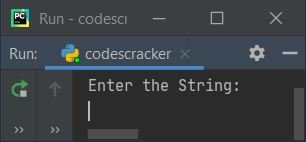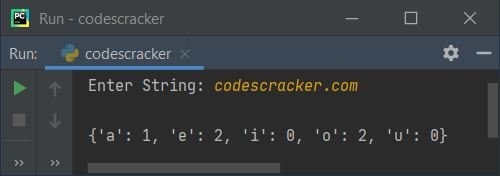# Python Program to Count Number of Each Vowels in String

This article is created to cover some programs in Python, that count and prints number of each vowels in a string entered by user. Here are the list of approaches used:

• Count number of each vowels in a string using, for loop and if-elif statements
• Using list
• Using dictionary

## Count Each Vowels using for Loop and if-else

This program count and prints number of each vowels present in a string, using for loop and if-elif statements. The question is, write a Python program to count number of each vowels. Here is its answer:

```print("Enter the String:")
text = input()

vowela = ['a', 'A']
vowele = ['e', 'E']
voweli = ['i', 'I']
vowelo = ['o', 'O']
vowelu = ['u', 'U']
ca = 0
ce = 0
ci = 0
co = 0
cu = 0

for x in text:
if x in vowela:
ca = ca+1
elif x in vowele:
ce = ce+1
elif x in voweli:
ci = ci+1
elif x in vowelo:
co = co+1
elif x in vowelu:
cu = cu+1

print("\n'a' occurs ", ca)
print("'e' occurs ", ce)
print("'i' occurs ", ci)
print("'o' occurs ", co)
print("'u' occurs ", cu)```

This program produces the following output:Now supply the input say Welcome to codescracker.com as string, then press `ENTER` key to count and print number of each vowels in given string as shown in the snapshot given below:The dry run of above program with same user input as provided in above sample run, goes like:

• Initial values, text = "Welcome to codescracker.com" (entered by user), ca=0, ce=0, ci=0, co=0, cu=0
• Now the execution of for loop begins, that is, the following code:
`for x in text:`
states that, one by one, each characters gets copied to x. That is, at first, "W" (the very first character of text) gets copied to x, at second "e" gets copied to x, and so on upto the last character of text
• Inside the loop, the following code:
`if x in vowela:`
states that if value of x is available in the list named vowela, then the condition (of if) evaluates to be true, and program flow goes inside its body and the value of ca gets incremented by 1
• Therefore, at first evaluation of for loop, x="W". And "W" is not available in any of all five lists namely ca, ce, ci, co, and cu
• Therefore, x="e", the second character gets copied to x and again checks for matching
• This time, "e" is available in the list vowele, therefore the condition (of first elif) x in vowele or "e" in ['e', 'E'] evaluates to be true, therefore program flow goes inside this elif's body and ce+1 or 0+1 or 1 gets initialized to ce
• This process continues, until each and every characters gets scanned and checked
• In this way, all five vowels gets counted. So just print its value after exiting from the loop

#### Modified Version of Previous Program

This program is the modified version of previous. This program uses end to skip inserting an automatic newline using print()

```print("Enter String: ", end="")
text = input()

v = ['a', 'e', 'i', 'o', 'u', 'A', 'E', 'I', 'O', 'U']
va = ['a', 'A']
ve = ['e', 'E']
vi = ['i', 'I']
vo = ['o', 'O']
vu = ['u', 'U']
ca = 0
ce = 0
ci = 0
co = 0
cu = 0

for x in text:
if x in v:
if x in va:
ca += 1
elif x in ve:
ce += 1
elif x in vi:
ci += 1
elif x in vo:
co += 1
elif x in vu:
cu += 1

print()
if ca>0:
print("\"a\" occurs", ca, "times")
if ce>0:
print("\"e\" occurs", ce, "times")
if ci>0:
print("\"i\" occurs", ci, "times")
if co>0:
print("\"o\" occurs", co, "times")
if cu>0:
print("\"u\" occurs", cu, "times")```

Here is its sample run with user input, HellO, wElcomE tO cOdescrAckEr.cOm as string:Note - The \" is used to print "

## Count Number of Each Vowels using List

This program uses list to do the same job as of previous program. The list is used in a way that, at 0th index, frequency of 'a' gets stored, at 1st index, frequency of 'e' gets stored, and so on in sequence of a, e, i, o, u. The count refers to value (element) at 0th index of list named count.

```print("Enter String: ", end="")
text = input()

count = [0, 0, 0, 0, 0]
text = text.casefold()
vowels = ['a', 'e', 'i', 'o', 'u']

for ch in text:
if ch in vowels:
if ch=='a':
count += 1
elif ch=='e':
count += 1
elif ch=='i':
count += 1
elif ch=='o':
count += 1
elif ch=='u':
count += 1

print()
for i in range(len(count)):
if i==0:
if count[i]>0:
print("'a' occurs", count[i], "time(s)")
if i==1:
if count[i]>0:
print("'e' occurs", count[i], "time(s)")
if i==2:
if count[i]>0:
print("'i' occurs", count[i], "time(s)")
if i==3:
if count[i]>0:
print("'o' occurs", count[i], "time(s)")
if i==4:
if count[i]>0:
print("'u' occurs", count[i], "time(s)")```

Here is its sample run with codescracker.com as string input:Note - The casefold() method is used to convert whole string passed as its argument, to lowercase.

## Count Each Vowels using Dictionary

This is the last program of this article, uses dictionary to count number of each vowel in a string entered by user at run-time. Dictionary is used to store information in key:value pairs

```print("Enter String: ", end="")
text = input()

vowels = ['a', 'e', 'i', 'o', 'u']
text = text.casefold()
count = {}.fromkeys(vowels, 0)

print()

for ch in text:
if ch in count:
count[ch] += 1

print(count)```

Here is its sample run with user input as of previous program's sample run:Note - Dictionary are defined using {} (curly) braces.

Note - The fromkeys() method returns dictionary with specified keys and value. That is, the first argument refers to keys, whereas the second argument refers to a value.

The following statement (from above program):

`count[ch] += 1`

fetches value with specified key, that is ch and increments the value by 1.

Python Online Test

« Previous Program Next Program »